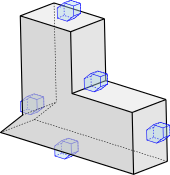# Higher-dimensional straight skeletons

Straight skeletons are thoroughly studied in the plane, both in theory and practice. There are also several papers on weighted straight skeletons in the plane, and the meanwhile a rigorous definition has been established. Interestingly, a generalization of straight skeletons to dimensions three or higher appears to be particularily hard. A main difficulity already lies in a proper definition of the combinatorial structure of the intiial wavefront of a three-dimensional polyhedra at vertices where no ball exists that is tangent to all incident faces, see the papers by Aurenhammer et al.1 and Barequet et al.2.

Straight skeletons in $$\mathbb{R}^d$$. In [Hub*14] we avoid the previous difficulties by considering Voronoi diagrams under polyhedral distance functions in order to define straight skeletons in $$\mathbb{R}^d$$ with arbitrary $$d \ge 2$$.

The idea is that straight skeletons in the plane are defined by a so-called wavefront propagation process. However, also Voronoi diagrams under convex distance functions can be defined this way. Moreover, for certain convex distance functions there is a class of input polygons where the straight skeleton and the Voronoi diagram coincide. In [Hub*14] we completely classify all such convex distance functions and all such input. (We call such input polyhedra “proper”.) Furthermore, we use this approach to generalize straight skeletons to arbitrary dimensions for “proper” polyhedra w.r.t. a proper distance function. We also show how an arbitrary input polyhedron can be approximated by a proper polyhedron w.r.t. a proper distance function.1. F. Aurenhammer and G. Walzl. Structure and computation of straight skeletons in 3-space. In Lecture Notes in Computer Science (LNCS), Proc. 24th International Symposium on Algorithms and Computation (ISAAC 2013), pages 44–54, Hong Kong, 2013.

2. G. Barequet, D. Eppstein, M. T. Goodrich, and A. Vaxman. Straight skeletons of three-dimensional polyhedra. CCCG 2014, Halifax, Nova Scotia, August 11–13, 2013 In Lecture Notes in Computer Science (LNCS), Proc. 16th European Symposium on Algorithms (ESA 2008), volume 5193, pages 148–160, 2008.